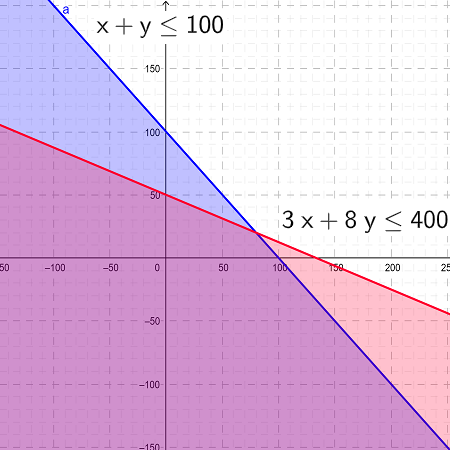# A company produces doll houses and sets of doll furniture. The doll houses take 3 hours of labor...

## Question:

A company produces doll houses and sets of doll furniture. The doll houses take 3 hours of labor to produce, and the furniture sets take 8 hours. The labor available is limited to 400 hours per week, and the total production capacity is 100 items per week. Existing orders require that at least 20 doll houses and 10 sets of furniture be produced per week. Write a system of inequalities representing this situation, where {eq}x {/eq} is the number of doll houses and {eq}y {/eq} is the number of furniture sets.

## System of linear inequalities

A system of linear inequalities of two variables consists of two or more linear inequalities with two same variables. These inequalities represent a range of possible values of variables of the inequalities

These can be solved simultaneously and by plotting it on a graph to get an acceptable range of values.

Let us assume that the number of dollhouses produced be {eq}x {/eq} and the number of furniture produced is {eq}y. {/eq}

According to the question, the dollhouses take 3 hours of labor to produce, and the furniture sets take 8 hours. The labor available is limited to 400 hours per week.

$$3x + 8y \le 400$$

According to the question, the total production capacity is 100 items per week.

$$x + y \le 100$$

Solving the two equations simultaneously.

$$(3x + 8y \le 400) - 3*(x + y \le 100)$$

$$5y \le 100$$

$$y \le 20$$

The minimum number of furniture set is 10, for this value the maximum number of dollhouses manufactured can be calculated.

Using the value of {eq}y=10 {/eq} in the equaiton {eq}x + y \le 100. {/eq}

$$x + y \le 100$$

$$x + 10 \le 100$$

$$x \le 90$$

The number of dollhouses and furniture manufactures is less than or equal to {eq}90\ and\ 20 {/eq} respectively.

The system of inequalities are {eq}3x + 8y \le 400 {/eq} and {eq}x + y \le 100 {/eq} and shown in the graph below in red and blue color respectively.